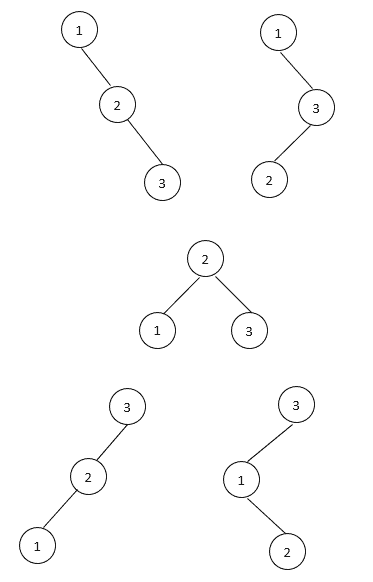## 1. Introduction

A binary tree is a hierarchical data structure in which each node has at most two children. Also, a binary search tree (BST) is a more specific type of binary tree where the main characteristic is that it’s sorted. Therefore, a binary tree is a BST if and only if an in-order traversal of the binary tree results in a sorted sequence.

This tutorial will show how to compute the number of binary search trees based on the number of tree nodes.

## 2. Unique Number of Binary Search Trees

In a BST, each node contains a sortable key. For example, we can label each node with an integer number. Therefore, the key in each node of a BST is greater than or equal to any key stored in the left subtree and less than or equal to any key stored in the right subtree.

Given a list ofdistinct numbers, we are interested in computing the number of BSTs labeled by these numbers. Without the loss of generality, we can assume the numbers are. For example, there are 5 possible binary search trees for:Although it is easy to enumerate all possible tree structures whenis small, the number of unique BST structures increases very fast whengets bigger. For example, there areunique BST structures when. Therefore, we need to compute this number with an algorithm. In this tutorial, we’ll show different approaches to compute this number.

## 3. Recursive Approach

Letdenote the total number of unique BST structures for number. For a list ofunique numbers, we can choose any number as the root. For example, we can choose the number() as the root. Then, numbers inwill be in the left subtree. Also, numbers inwill be in the right subtree.

Since we havenumbers in the left subtree, the left subtree hasunique BST structures. Similarly, the right subtree hasunique tree structures. Also, the arrangements in the left and right subtrees are independent. Therefore, we have a total ofunique tree structures whenis the root.

Since we havepossible ways to choose the root, we can add togetherfor all possible values of:If we don’t have any nodes in one subtree, then we’ll only consider the total number of unique tree structures in the other subtree. Therefore, the base case of the formula is.

As a result, we can construct a recursive algorithm based on the above formula:## 4. Dynamic Programming Approach

In general, the recursive algorithm is straightforward based on the recursive formula. However, it has many repeat computations. To avoid that, we can use a dynamic programming approach to compute the number.

We can first computewith smaller numbers and store the results into an array. Then, when we compute bigger numbers, we can reuse the previously computed results from the smaller numbers:In this algorithm, we use a loop to compute our number step by step. For a number, we use another loop to compute its value based on our recursive formula.

For example, our base case is. Then, we computebased on the value of. After that, the next step is to computebased on the values ofand.

We repeat this process for each number from 1 to. Therefore, when we compute, all the required values for the computation are already available. Eventually, we’ll computeand return this value.

Since we have a nested loop to do the computation, the overall time complexity of this algorithm is.

## 5. Catalan Number Approach

The number of different BSTs is actually a Catalan Number:Therefore, we can compute our numberdirectly from the Catalan Number. However, we need to compute 3 factorial numbers based on this formula.

To avoid the time consuming factorial computations, we can also use the following recurrence property of Catalan Number in our algorithm:As a result, the algorithm based on this recurrence is:Since we use only one loop to do the computation, the overall running time is.

## 6. Conclusion

This tutorial showed a recursive formula to compute the number of unique binary search trees withnodes. Also, we provided several approaches to compute this number. As a result, the Catalan Number approach is the fastest algorithm among them to compute this number. However, the dynamic programming approach is more intuitive as it directly comes from the analysis of BST structures.